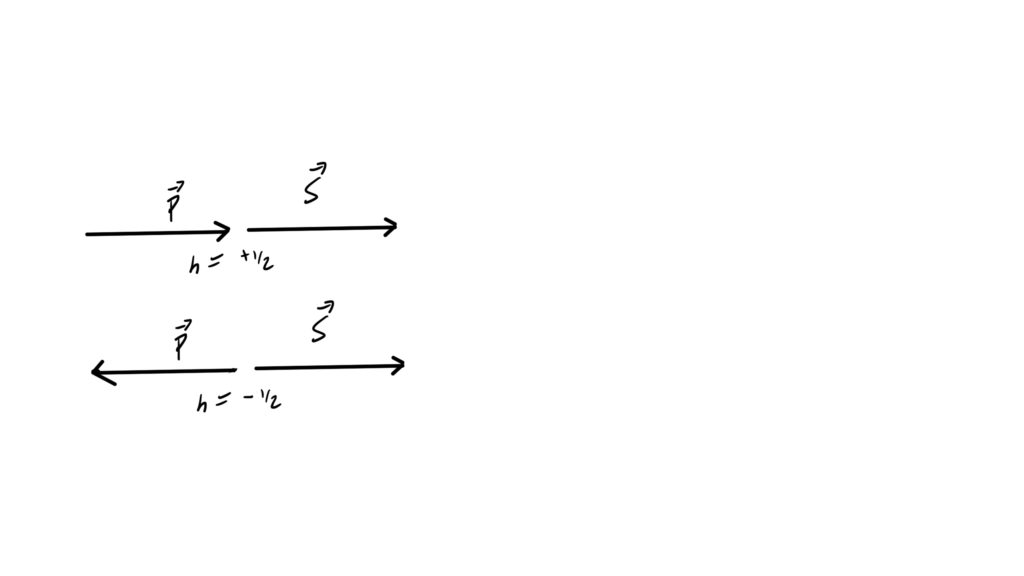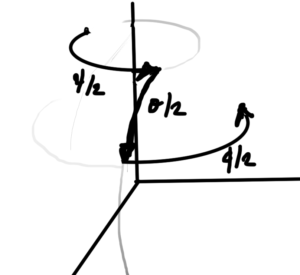## PHY2403H Quantum Field Theory. Lecture 21, Part I: Dirac equation solutions, orthogonality conditions, direct products. Taught by Prof. Erich Poppitz

### DISCLAIMER: Rough notes from class, with some additional side notes.

These are notes for the UofT course PHY2403H, Quantum Field Theory, taught by Prof. Erich Poppitz, fall 2018.

## Overview.

See the PDF above for full notes for the first part of this particular lecture. We covered

• Normalization:
\begin{equation*}
u^{r \dagger} u^{s}
= 2 p_0 \delta^{r s}.
\end{equation*}
• Products of $$p \cdot \sigma, p \cdot \overline{\sigma}$$
\begin{equation*}
(p \cdot \sigma) (p \cdot \overline{\sigma})
=
(p \cdot \overline{\sigma}) (p \cdot \sigma)
= m^2.
\end{equation*}
• Adjoint orthogonality conditions for $$u$$
\begin{equation*}
\overline{u}^r(\Bp) u^{s}(\Bp) = 2 m \delta^{r s}.
\end{equation*}
• Solutions in the $$e^{i p \cdot x}$$ “direction”
\begin{equation}\label{eqn:qftLecture21:99}
v^s(p)
=
\begin{bmatrix}
\sqrt{p \cdot \sigma} \eta^s \\
-\sqrt{p \cdot \overline{\sigma}} \eta^s \\
\end{bmatrix},
\end{equation}
where $$\eta^1 = (1,0)^\T, \eta^2 = (0,1)^\T$$.
• $$v$$ normalization
\begin{equation*}
\begin{aligned}
\overline{v}^r(p) v^s(p) &= – 2 m \delta^{rs} \\
v^{r \dagger}(p) v^s(p) &= 2 p^0 \delta^{rs}.
\end{aligned}
\end{equation*}
\begin{equation*}
\begin{aligned}
\overline{u}^r(p) v^s(p) &= 0 \\
\overline{v}^r(p) u^s(p) &= 0.
\end{aligned}
\end{equation*}
• Dagger orthogonality conditions.
\begin{equation*}
\begin{aligned}
v^{r \dagger}(-\Bp) u^s(\Bp) &= 0 \\
u^{r\dagger}(\Bp) v^s(-\Bp) &= 0.
\end{aligned}
\end{equation*}
• Tensor product.

Given a pair of vectors
\begin{equation*}
x =
\begin{bmatrix}
x_1 \\
\vdots \\
x_n \\
\end{bmatrix},
y =
\begin{bmatrix}
y_1 \\
\vdots \\
y_n \\
\end{bmatrix},
\end{equation*}
the tensor product is the matrix of all elements $$x_i y_j$$

\begin{equation*}
x \otimes y^\T =
\begin{bmatrix}
x_1 \\
\vdots \\
x_n \\
\end{bmatrix}
\otimes
\begin{bmatrix}
y_1 \cdots y_n
\end{bmatrix}
=
\begin{bmatrix}
x_1 y_1 & x_1 y_2 & \cdots & x_1 y_n \\
x_2 y_1 & x_2 y_2 & \cdots & x_2 y_n \\
x_3 y_1 & \ddots & & \\
\vdots & & & \\
x_n y_1 & \cdots & & x_n y_n
\end{bmatrix}.
\end{equation*}

• Direct product relations.
\begin{equation*}
\begin{aligned}
\sum_{s = 1}^2 u^s(p) \otimes \overline{u}^s(p) &= \gamma \cdot p + m \\
\sum_{s = 1}^2 v^s(p) \otimes \overline{v}^s(p) &= \gamma \cdot p – m \\
\end{aligned}
\end{equation*}

## PHY2403H Quantum Field Theory. Lecture 20: Dirac Lagrangian, spinor solutions to the KG equation, Dirac matrices, plane wave solution, helicity. Taught by Prof. Erich Poppitz

[Here is another PDF only post, containing my notes for Lecture 20 of the UofT QFT I (quantum field theory) course.]

In this lecture Professor Poppitz derived a rest frame solution of the Dirac equation, then demonstrated that the generalization to non-zero momentum satisfied the equation. We also saw that Dirac spinor solutions of the Dirac equation are KG equation solutions, and touched on the relation of some solutions to the helicity operator.This post doesn’t have a web version, since my latex -> wordpress-mathjax script doesn’t have support for the theorem/lemma environments that I used for Monday’s notes, and I don’t have time to implement that right now.

## PHY2403H Quantum Field Theory. Lecture 19: Pauli matrices, Weyl spinors, SL(2,c), Weyl action, Weyl equation, Dirac matrix, Dirac action, Dirac Lagrangian. Taught by Prof. Erich Poppitz

[Here are my notes for lecture 19 of the UofT course PHY2403H, Quantum Field Theory, taught by Prof. Erich Poppitz, fall 2018.] For this lecture my notes are pdf only, due to length. While the after-class length was 8 pages, it ended up expanded to 17 pages by the time I finished making sense of the material.These also include a portion of the notes from Lecture 18 (not yet posted), as it made sense to group all the Pauli matrix related content.  This particular set of notes diverges from the format presented in class, as it made sense to me to group things in this particular lecture in a more structured definition, theorem, proof style.  I’ve added a number of additional details that I found helpful, as well as a couple of extra problems (some set as formal problems at the end, and others set as theorem or lemmas in with the rest.)

## Reflection using Pauli matrices.

November 22, 2018 phy2403 , , , , ,

In class yesterday (lecture 19, notes not yet posted) we used $$\Bsigma^\T = -\sigma_2 \Bsigma \sigma_2$$, which implicitly shows that $$(\Bsigma \cdot \Bx)^\T$$ is a reflection about the y-axis.
This form of reflection will be familiar to a student of geometric algebra (see  — a great book, one copy of which is in the physics library). I can’t recall any mention of the geometrical reflection identity from when I took QM. It’s a fun exercise to demonstrate the reflection identity when constrained to the Pauli matrix notation.

## Theorem: Reflection about a normal.

Given a unit vector $$\ncap \in \mathbb{R}^3$$ and a vector $$\Bx \in \mathbb{R}^3$$ the reflection of $$\Bx$$ about a plane with normal $$\ncap$$ can be represented in Pauli notation as
\begin{equation*}
-\Bsigma \cdot \ncap \Bsigma \cdot \Bx \Bsigma \cdot \ncap.
\end{equation*}

To prove this, first note that in standard vector notation, we can decompose a vector into its projective and rejective components
\begin{equation}\label{eqn:reflection:20}
\Bx = (\Bx \cdot \ncap) \ncap + \lr{ \Bx – (\Bx \cdot \ncap) \ncap }.
\end{equation}
A reflection about the plane normal to $$\ncap$$ just flips the component in the direction of $$\ncap$$, leaving the rest unchanged. That is
\begin{equation}\label{eqn:reflection:40}
-(\Bx \cdot \ncap) \ncap + \lr{ \Bx – (\Bx \cdot \ncap) \ncap }
=
\Bx – 2 (\Bx \cdot \ncap) \ncap.
\end{equation}
We may write this in $$\Bsigma$$ notation as
\begin{equation}\label{eqn:reflection:60}
\Bsigma \cdot \Bx – 2 \Bx \cdot \ncap \Bsigma \cdot \ncap.
\end{equation}
We also know that
\begin{equation}\label{eqn:reflection:80}
\begin{aligned}
\Bsigma \cdot \Ba \Bsigma \cdot \Bb &= a \cdot b + i \Bsigma \cdot (\Ba \cross \Bb) \\
\Bsigma \cdot \Bb \Bsigma \cdot \Ba &= a \cdot b – i \Bsigma \cdot (\Ba \cross \Bb),
\end{aligned}
\end{equation}
or
\begin{equation}\label{eqn:reflection:100}
a \cdot b = \inv{2} \symmetric{\Bsigma \cdot \Ba}{\Bsigma \cdot \Bb},
\end{equation}
where $$\symmetric{\Ba}{\Bb}$$ is the anticommutator of $$\Ba, \Bb$$.
Inserting \ref{eqn:reflection:100} into \ref{eqn:reflection:60} we find that the reflection is
\begin{equation}\label{eqn:reflection:120}
\begin{aligned}
\Bsigma \cdot \Bx –
\symmetric{\Bsigma \cdot \ncap}{\Bsigma \cdot \Bx}
\Bsigma \cdot \ncap
&=
\Bsigma \cdot \Bx –
{\Bsigma \cdot \ncap}{\Bsigma \cdot \Bx}
\Bsigma \cdot \ncap

{\Bsigma \cdot \Bx}{\Bsigma \cdot \ncap}
\Bsigma \cdot \ncap \\
&=
\Bsigma \cdot \Bx –
{\Bsigma \cdot \ncap}{\Bsigma \cdot \Bx}
\Bsigma \cdot \ncap

{\Bsigma \cdot \Bx} \\
&=

{\Bsigma \cdot \ncap}{\Bsigma \cdot \Bx}
\Bsigma \cdot \ncap,
\end{aligned}
\end{equation}
which completes the proof.

When we expand $$(\Bsigma \cdot \Bx)^\T$$ and find
\begin{equation}\label{eqn:reflection:n}
(\Bsigma \cdot \Bx)^\T
=
\sigma^1 x^1 – \sigma^2 x^2 + \sigma^3 x^3,
\end{equation}
it is clear that this coordinate expansion is a reflection about the y-axis. Knowing the reflection formula above provides a rationale for why we might want to write this in the compact form $$-\sigma^2 (\Bsigma \cdot \Bx) \sigma^2$$, which might not be obvious otherwise.

# References

 C. Doran and A.N. Lasenby. Geometric algebra for physicists. Cambridge University Press New York, Cambridge, UK, 1st edition, 2003.

## my course evaluation comments for PHY2403 — Quantum Field Theory I.

November 20, 2018 phy2403 , , ,

Here are my evaluation comments for QFT I. The university provides an anonymous facility to submit course feedback, but since I have no conflicts that require anonymization, I’m posting my commentary (and rationale for some of my list selections) publicly.

## Q) Please comment on the overall quality of the instruction in this course.

Professor Poppitz’s knowledge of the subject matter is impressive and thorough. I expect that this is a particularly difficult course to teach and think that he has done an admirable job trying to work through the maximum amount of material in the limited time available in this course.

It is challenging but fun game (albeit a slightly masochistic one) to keep up with Prof Poppitz’s blistering pace through the course material. Poppitz often says “Phew!!” at the end of the race to complete a long derivation in the allotted time, and I’ll be saying the same thing at the end of this course.

The barrage of abstract material covered in a lecture is often sufficient to leave me with a headache, and it takes a few hours to recover from each class. It takes a few more hours after that to digest the material at a human pace.

This course would strongly benefit from video recorded lectures that some of the more educationally progressive academic institutions currently provide (i.e. MIT’s OCW and Yale’s “Open Yale” courses). With the exception of the UofT SciNet group (phy1610 — Scientific computing for Physicists), I’m not aware of any UofT physics courses that provide such recordings. For phy2403, video recordings would be particularly valuable, as it would allow the student to “pause” the Professor and work through the material presented at an individually suitable pace.

## Q) Please comment on any assistance that was available to support your learning in the course.

Prof Poppitz was available continually on the course forum, after class briefly, and in weekly office hours. I received a great deal of helpful assistance from him during the course.

## Q) Compared to other courses, the workload for this course was…

I picked Heavy (not Very Heavy), but I’m not in a good position to evaluate since I’m only taking one course.

## Q) I would recommend this course to other students.

I picked Mostly (not Strongly). I wouldn’t recommend this course to anybody who was not adequately prepared. I’m not sure that I was. It is a very tough course. I was continually impressed with the other students in the class. I’ve worked slowly for years to gradually build up the background required to take this course, and all the rest of these younglings are downing the material with seeming ease. There are a lot of exceptionally smart students enrolled on this course.